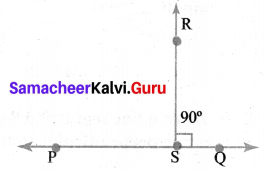## Tamilnadu Samacheer Kalvi 6th Maths Solutions Term 2 Chapter 4 Geometry Additional Questions

Question 1.
Name the type of the following triangles.
(a) ∆PQR with m∠Q = 90°
(b) ∆ABC with m∠B = 90° and AB = BC
Solution:
(a) One of the angles is 90°
It is a right-angled triangle
(b) Since two sides are equal.
It is an isosceles triangle. Also m∠B = 90°
It is an Isosceles right-angled triangle

Question 2.
Classify the triangles (scalene, isosceles, equilateral) given below.
(a) ∆ABC, AB = BC
(b) ∆PQR, PQ = QR = RP
(c) ∆ABC, ∠B = 90°
(d) ∆EFG, EF = 3 cm, FG = 4 cm and GE = 3 cm
Solution:
(a) Isosceles triangle
(b) Equilateral triangle
(c) Right angled triangle
(d) Isosceles triangle

Question 3.
In triangle ∆ABC, AB = BC = CA = 5 cm. Then what is the value of ∠A, ∠B and ∠C?
Solution:
Since AB = BC = CA
∠A = ∠B = ∠C
We know that ∠A + ∠B + ∠C = 180°
∴ ∠A = ∠B = ∠C = 60°

Question 4.
In ∆PQR, ∠P = ∠Q = ∠R = 60°, then what can you say about the length of sides of ∆PQR? Also, write the name of the triangle?
Solution:
∠P = ∠Q = ∠R = 60°
So PQ = QR = RP
∆PQR is equilateral triangle

Question 5.
In ∆ABC, AB = BC and ∠A = 50°. Then find the value of ∠C?
Solution: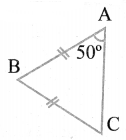It is an isosceles triangle.
∠A = ∠C
∠C = 50°Question 6.
(a) Try to construct triangles using matchsticks.
(b) Can you make a triangle with?
(i) 3 matchsticks?
(ii) 4 matchsticks?
(iii) 5 matchsticks?
(iv) 6 matchsticks?
Name the type of triangle in each case. If you cannot make a triangle think of the reason for it.
Solution: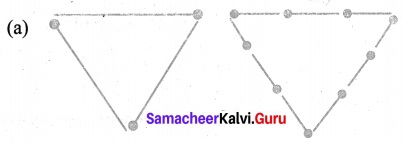(b) (i) With the help of 3 matchsticks, we can make an equilateral triangle. Since all three matchsticks are of equal length.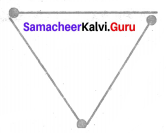(ii) With the help of 4 matchsticks, we cannot make any triangle because in this case, sum of two sides is equal to the third side and we know that the sum of the lengths of any two sides of a triangle is always greater than the length of the third side.
(iii) With the help of 5 matchsticks, we can make an isosceles triangle. Since we get two sides equal in this case.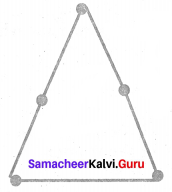(iv) With the help of 6 matchsticks, we can make an equilateral triangle. Since we get three sides equal in length.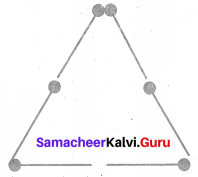Question 7.
A table is bought for ₹ 4500 and sold for ₹ 4800. Find the profit or loss.
Solution:
C.P = ₹ 4500
S.P. = ₹ 4800
Here S.P< C.P
Profit = S.P – C.P = ₹ 4800 – ₹ 4500 = ₹ 300Question 8.
Draw any line segment $$\overline{\mathbf{P Q}}$$. Take any point R not on it. Through R, draw a perpendicular to $$\overline{\mathbf{P Q}}$$.
Solution:
Construction:
(i) Drawn a line segment $$\overline{\mathbf{P Q}}$$ using scale and taken a point R outside of $$\overline{\mathbf{P Q}}$$.
(ii) Placed a set-square on $$\overline{\mathbf{P Q}}$$ such that one arm of its right angle aligns along $$\overline{\mathbf{P Q}}$$.
(iii) Placed a scale along the other edge of the right angle of the set-square
(iv) Slide the set-square along the line till the point R touches the other arm of its right angle.
(v) Joined RS along the edge through R meeting $$\overline{\mathbf{P Q}}$$ at S.
Hence $$\overline{\mathbf{R S}}$$ ⊥ $$\overline{\mathbf{P Q}}$$.